Custom math worksheets at your fingertips# Details for problem "Add numbers"

Quickname: 4897

Elementary School, Primary School, Junior High School, Middle School.

## Summary

Numbers with or without decimal places have to be added.

## Examples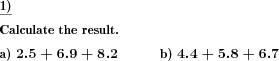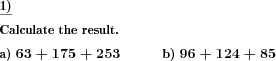## Description

The addition is the first arithmetic operation taught right after the identification of numbers. In the problem, a series of positive numbers are presented. The task is to determine the sum of these numbers by adding them up. The range of the numbers can be chosen. All the numbers and the sum will be in that range.

Numbers may be selected to be all whole numbers or have a specified number of decimal places, so they are decimal fractions.

This problem offers a great level of flexibility and customized problems generated can be used for grade 1 to grade 6.

Exercising these tasks will offer an expansion of the following skills.

1. Revision of addition concepts with or without carries.
2. Horizontal addition of multiple numbers.
3. The use of decimal points as a worksheet may be customized for the addition of two or more decimal fractions.
4. Develop a concept of more complex addition problems with multiple summands consisting of multiple digits.
5. These worksheets offer the addition of decimal fractions which lays a foundation for real-world problems.

The following customizaton options are available.

• The number of problems can range from 1 to 10 for each particular skill level.
• The number of summands can vary from 2 to 8.
• The selection of numbers starts from 20 to 100,000.
• The decimal part is also selectable from 0 to 3.

The answer key will provide the sum of all numbers for all problems.

Download free printable worksheets for this math problem here. The worksheet contains the problems only, the solution sheet includes the answers. Just click on the respective link.

•Worksheet 1Solution sheet with answers
•Worksheet 2Solution sheet with answers
•Worksheet 3Solution sheet with answers

If you can not see the solution sheets for download, they may be filtered out by an ad blocker that you may have installed. If this is the case, please allow ads for this page and reload the page. The solution sheets will then reappear.

• Do these sample worksheets do not really fit?
• Do you need more math worksheets, with a different level of difficulty?
• Would you like to combine different problems on a worksheet and adjust them to your needs?
• As a teacher, you can put together your own worksheets using the automatically generated math problems provided.
With a free initial credit, you can start creating your own math worksheets in a few minutes.

You can try it for free! Register here, to create custom worksheets now!

## Customization options for this problem

Parameter
Possible values
Number of problems
1, 2, 3, 4, 5, 6, 7, 8, 9, 10
Number of summands
2, 3, 4, 5, 6, 7, 8
Number range
20, 30, 40, 50, 100, 200, 500, 1000, 10000, 100000
Decimals
0, 1, 2, 3

## Similar problems

Remark
Description
With multiples of powers of ten
Multiples of powers of ten added to natural numbers.
If you want to explicitely allow or forbid carries
Addition of two numbers with controlled number of digits for each number.
Long addition of numbers with decimals
Columnar addition of multiple decimal fractions.
As a frames and arrows style calculation chain, successive application the same operation
Simple frames and arrows chain task with one elementary arithmetic operation, results are to be filled in blank spaces.
A fill-in problem: Frames and arrows
In a frames and arrows calculation chain task, the results are to be filled in the blank spaces.

## Other types of problems that appear on worksheets with this problem:

Relevance
Name
Description
Quickname
Example
****
Add and subtract spliting one digit number
Addition and subtraction by splitting one digit number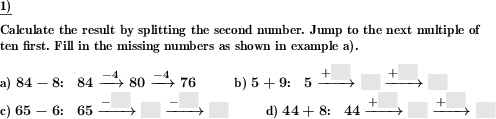****
Add multiples of powers of ten
Multiples of powers of ten added to natural numbers.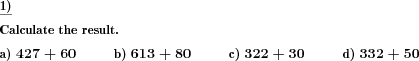****
Addition of powers of ten, fill blanks
What multiple of a power of ten has been added?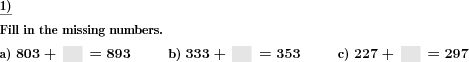****
Determine shapes with same shaded fraction
In a series of shapes find the two shapes that represent the same fraction.